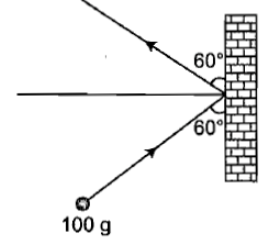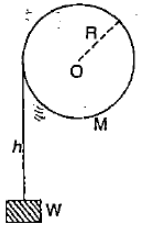A wheel whose moment of inertia is 12 ${\mathrm{Kgm}}^{2}$ has an initial angular velocity of 40 rad/sec. A constant torque of 20 Nm acts on the wheel. The time in which the wheel is accelerated to 100 rad/sec is

1.  72 seconds

2.  16 seconds

3.  8 seconds

4.  36 seconds

Concept Questions :-

Torque
High Yielding Test Series + Question Bank - NEET 2020

Difficulty Level:

A flywheel is in the form of a uniform circular disc of radius 1 m and mass 2 kg. The work which must be done on it to increase its frequency of rotation from 5 rev ${\mathrm{s}}^{-1}$ to 10 rev ${\mathrm{s}}^{-1}$ is approximately

1.  1.5 x ${10}^{2}$ J

2.  3.0 x ${10}^{2}$ J

3.  1.5 x ${10}^{3}$ J

4.  3.0 x ${10}^{3}$ J

Concept Questions :-

Angular momentum
High Yielding Test Series + Question Bank - NEET 2020

Difficulty Level:

A particle of mass m has momentum p. it's kinetic energy will be

1.  mp

2.  ${p}^{2}m$

3.  $\frac{{p}^{2}}{m}$

4.  $\frac{{p}^{2}}{2m}$

Concept Questions :-

Linear momentum
High Yielding Test Series + Question Bank - NEET 2020

Difficulty Level:

A constant torque acting on a uniform circular wheel changes its angular momentum from ${\mathrm{A}}_{0}$ to  in 4s. The magnitude of this torque is

1. $\frac{3{\mathrm{A}}_{0}}{4}$

2.

3. ${\mathrm{A}}_{0}$

4.

Concept Questions :-

Angular momentum
High Yielding Test Series + Question Bank - NEET 2020

Difficulty Level:

Two discs are rotating about their axes, normal to the discs and passing through the centres of the discs. Disc D${}_{1}$ has 2 kg mass and 0.2 m radius and initial angular velocity of 50 rad s${}^{-1}$. Disc D${}_{2}$ has 4 kg mass, 0.1 m radius and initial angular velocity of 200 rad s${}^{-1}$. The two discs are brought in contact face to face, with their axes of rotation coincident. The final angular velocity (in rad.s${}^{-1}$) of the system is

1. 60

2. 100

3. 120

4. 40

Concept Questions :-

Angular momentum
High Yielding Test Series + Question Bank - NEET 2020

Difficulty Level:

A body rolls down an inclined plane without slipping. The fraction of total energy associated with its rotation will be

Where k is radius of gyration of the body about an axis passing through centre of mass and R is the radius of the body.

Concept Questions :-

Rolling motion
High Yielding Test Series + Question Bank - NEET 2020

Difficulty Level:

A mass of 100 g strikes the wall with speed 5 m/s at an angle as shown in figure and it rebounds with the same speed. If the contact time is $2×{10}^{-3}$ s, what is the force applied on the mass by the wall(1) N to right

(2) 250 N to right

(3) $250\sqrt{3}$ N to left

(4) 250 N to left

Concept Questions :-

Linear momentum
High Yielding Test Series + Question Bank - NEET 2020

Difficulty Level:

In the following figure, a weight W is attached to a string wrapped round a solid cylinder of mass M mounted on a frictionless horizontal axle at O.

If the weight starts from rest and falls a distance h, then its speed at that instant is(1) Proportional to R

(2) Proportional to $\frac{1}{R}$

(3) Proportional to $\frac{1}{{R}^{2}}$

(4) Independent of R

Concept Questions :-

Torque
High Yielding Test Series + Question Bank - NEET 2020

Difficulty Level:

A solid cylinder rolls down an inclined plane that has friction sufficient to prevent sliding. The ratio of rotational energy to total kinetic energy is

1.  $\frac{1}{2}$

2.  $\frac{1}{3}$

3.  $\frac{2}{3}$

4.  $\frac{3}{4}$

Concept Questions :-

Rolling motion
High Yielding Test Series + Question Bank - NEET 2020

Difficulty Level:

A swimmer while jumping into water from a height easily forms a loop in the air, if

1. He pulls his arms and legs in

2. He spreads his arms and legs

3. He keeps himself straight

4. None of the above

Concept Questions :-

Angular momentum